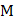# A rope ladder with a lengthcarrying a man with a massat its end is attached to the basket of balloon with a mass. The entire system is in equilibrium in the air. As the man climbs up the ladder into the balloon, the balloon descends b y a height.  Then the potential energy of the man a) Increase byb) Increase byc) Increases byd) Increases by## Question ID - 51042 :- A rope ladder with a lengthcarrying a man with a massat its end is attached to the basket of balloon with a mass. The entire system is in equilibrium in the air. As the man climbs up the ladder into the balloon, the balloon descends b y a height.  Then the potential energy of the man a) Increase byb) Increase byc) Increases byd) Increases by3537

 (a) Effective height through which man moves upNext Question :

The forceacting on a particle moving in a straight line is shown in figure. What is the work done by the force on the particle in the 1st meter of the trajectorya)b)c)d)